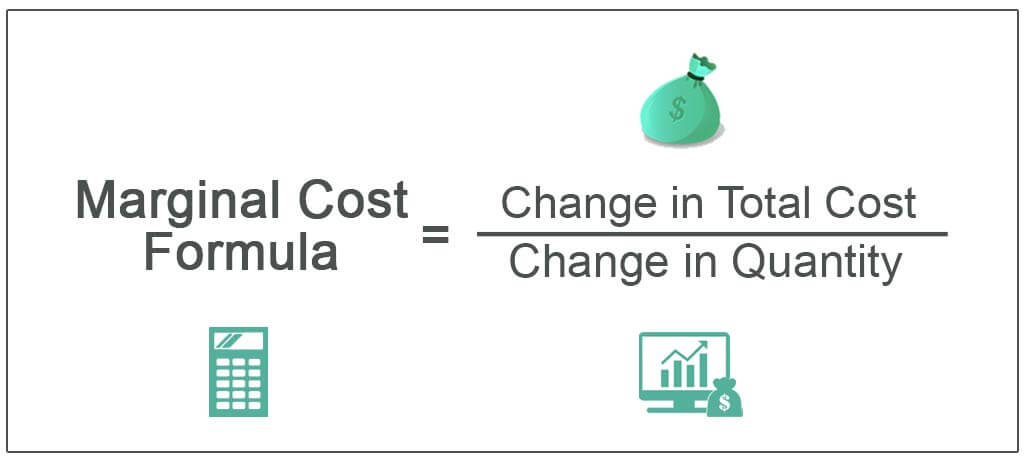# Marginal cost: Definition, formulas, curves and more

### Marginal cost definition

With the increase in one unit of output, the total cost increases, and the total increased unit is the marginal cost. Moreover, marginal cost is a change in total cost by producing an additional unit of output. Suppose we produce five units which cost 150 rupees. Thus, when we produce six units, the cost will be 160 rupees. So the marginal cost will be 10 rupees.### Marginal cost meaning

Normally cost means payment, expense etc. But for a manager economics person, cost means expenditure. Hence, marginal means an extra unit, and cost means the amount incurred in the production of a commodity. On the other hand, marginal cost means the amount incurred in the production of one additional unit.

### Marginal cost is also known as

The marginal cost’s other name is Incremental cost.

### Marginal cost PVR formula

However, the first and most important formula of marginal cost is profit volume ratio (PVR). PVR equals the contribution by sales into 100. Therefore, for two years, PVR equals to change in priority by a change in sales into 100.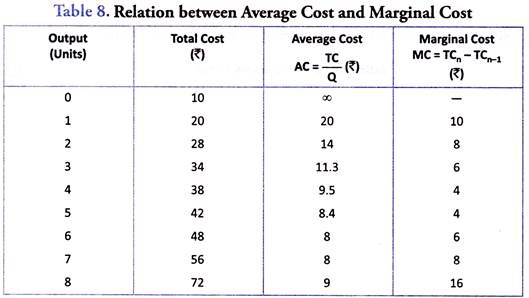### Marginal cost BEP formula

Moreover, the second formula of marginal cost is breakable point (BEP). Now, we need to find BEP in two ways. However, for rupees, we need to divide the fixed cost with the profit volume ratio. Hence, for breakable points in units, we need to divide the fixed cost (FC) with contribution per unit (CPU). Then, we need to take the denominator in per unit.

### Marginal cost MOS formula

Additionally, the third formula of marginal cost is margin of safety(MOS). Now, two formulas can calculate this formula. Therefore, to find the marginal safety of rupees, we need to divide profit with profit volume ratio. And to find the marginal safety of units, we need to divide profit with a contribution per unit. Thus, there is another formula of margin of safety, which is actual sales minus breakable points sales.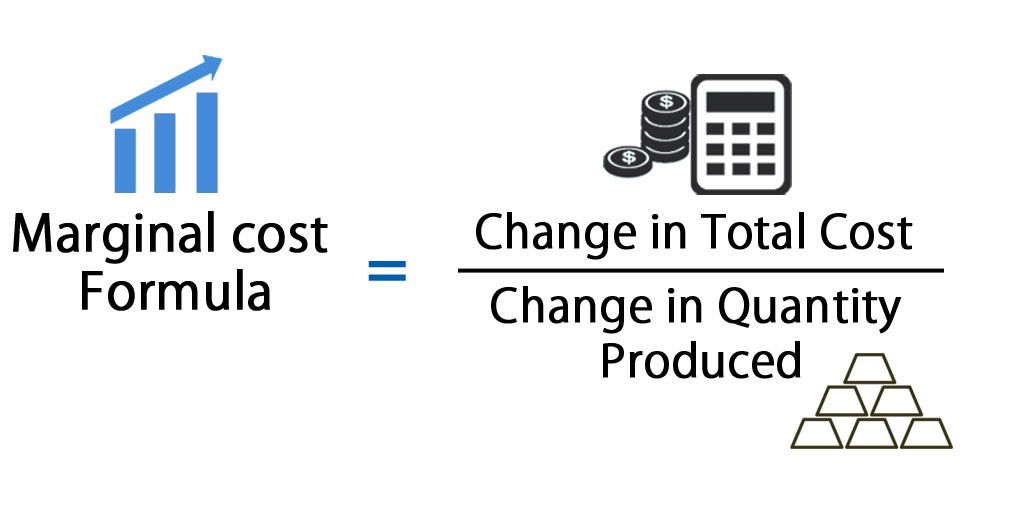### Marginal cost other formulas

If we need to calculate sales and have the profit information, the formula becomes required sales equal to fixed cost plus profit divided by profit volume ratio. To find the rupees, we need to take the profit volume ratio in the denominator. And to find the units, we need to take the contribution per unit in the denominator.

Whenever we need to calculate the profit, the formula will be sales multiplied by profit volume ratio minus fixed cost. Moreover, there are some questions like calculate sales in profit of two lakhs, calculate profit in sales of three lakhs. Usually, we should take sales which is respect to profit.

Next, the Fixed cost formula is sales into profit volume ratio minus profit. However, the fixed cost always remains fixed. If two different years are given in the questions, we only need to calculate the first year. So, we take only first-year sales and first-year profit.

However, when two years are given in the question, there is a formula to calculate this. Profit volume ratio equals to change in profit by a change in sales into 100. Thus, a change in profits means we need to take profits for two years. Also, we need to make sales of 2 years and multiply them by 100. At least we will get the result in percentage.

### Marginal cost curve

Now, the marginal cost curve is drawn with quantity on the horizontal axis and measurement of costs on the vertical axis. Next, a marginal cost curve, its shape from left to right, tracks the cost of each additional unit as we are increasing how many units the firm is producing. However, we can see that initially when the firm starts producing, the firm’s quantity is quite low. Moreover, the marginal costs initially decrease, meaning that each additional unit is cheaper to produce than the last. After some point, though, we see the marginal cost of each additional unit starts to become higher or more expensive than the last. Hence, this is when the marginal cost curve starts to increase and has the section that is positively sloped.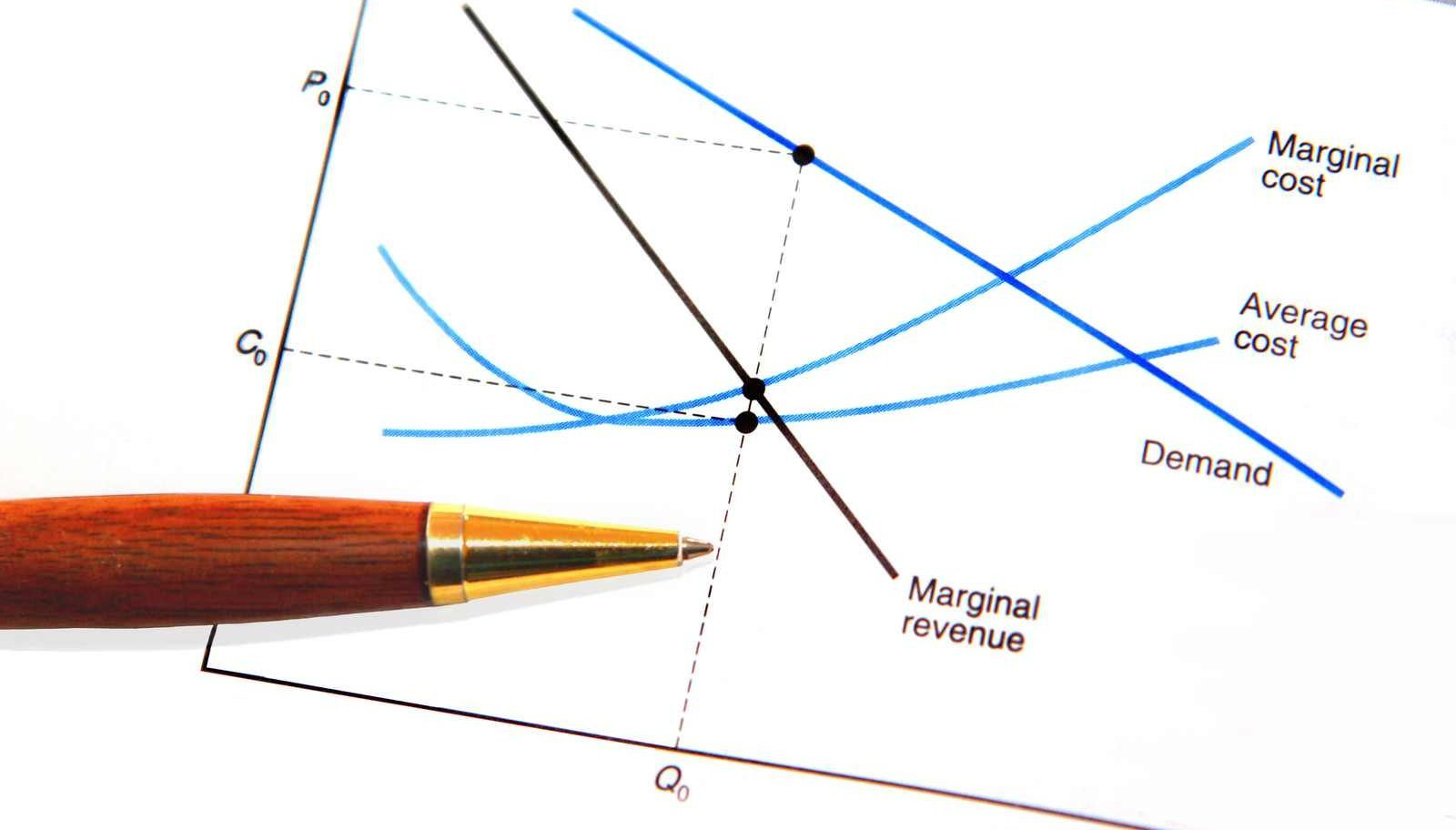However, there are some inputs capital, labor k, and L. So, a firm’s output Q, the quantity they are producing, depends on the level of capital k and labor L. Hence, the notation is the functional notation. Therefore, this entire means is that exactly the quantity produced by the firm is some function of the level of capital and labor. Moreover, the important thing is the fixed capital, so if a firm wants to increase its output once, you increase its Q. Hence, it can only change its level of labor; this is important if we want to produce more, we can only increase the amount of labor we are using. We can’t change our capital. Additionally, we are producing one level of a product, and we increase 2Q to two levels of production.

### Marginal cost pricing

However, marginal cost is equal to a change in the total cost upon a change in the quantity. Imagine there is a company whose name is x. Now, they produce 200 units of pens at the cost of 2000 rupees. So we can calculate the cost of 1 pen that is 2000 upon two hundred. So, the result is 10 rupees for each unit. Let us produce 201 additional units of pen except for 200 units. Next, that means we are going to produce 201 pens. Now, the Production cost will be two thousand plus ten, which equal two thousand ten rupees. Hence, the cost of the 201st unit is 10 rupees. However, the change in cost will be ten, and the unit’s change will be increased by 1. So, we put this in the formula. The result is ten upon one, which is equal to 10.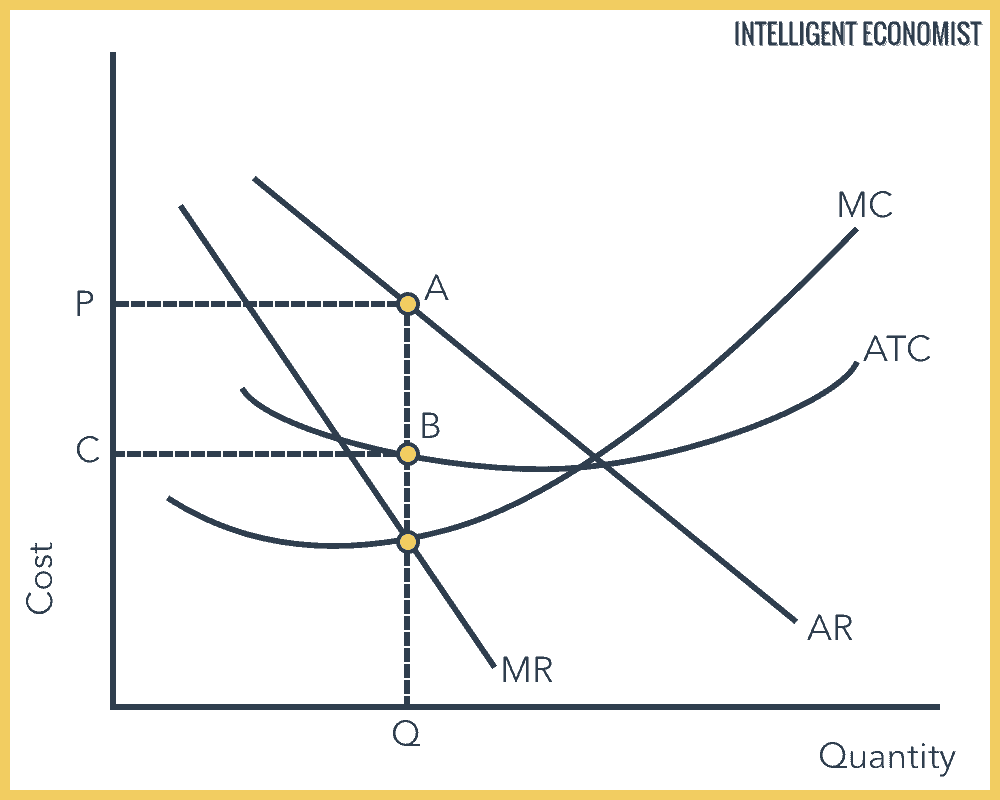### The marginal cost of capital

Moreover, the marginal cost of capital means the cost of raising an additional rupee. Next, that means we already have a balance that has share capitals, preference share, ventures and so on. If we need to increase the shares, then increasing the share is the marginal cost of capital. The formula of the marginal cost of capital is:

Cost of equity = Risk-free rate of return + Premium expected for risk.

Next, Cost of equity = Risk-free rate of return + Beta × (market rate of return – the risk-free rate of return).

1. The following information is provided regarding the specific cost of capital of different sources, along with the book value and market value weights.
 Source C/C BV MV Equity share capital 18% .50 .58 Preference share 15% .20 .17 Long term debts 7% .30 .25

Calculate the weighted average cost of capital, WACC, using both the BV and the MV weights.

1. The WACC based on BV and MV weights may be calculated as follows:
 Source BV MV C/C BV × C/C MV × C/C Equity share capital .50 .58 .18 .090 .1044 Preference shares .20 .17 .15 .030 .0255 Long term debt .30 .25 .07 .021 .0175 .141 .1474

The WACC based on BV weights is 14.1%, and

The WACC based on MV weights is 14.74%.

### Marginal cost and marginal benefit examples

Let’s say you are buying pizza by the slice. Now here’s the thing although you will pay the same price for every slice, whatever price they have listed. However, you are probably valuing them differently. So, let’s say the very first slice you buy and are willing to pay 100 rupees for that slice. So, that’s a high valuation, but then the second slice you buy, you don’t value it quite as high. Then, you are willing to pay seven hundred rupees for that one. And the third slice you buy and are willing to pay 200 rupees for that one. So, these numbers are marginal benefits. Hence, the benefit you get from the next slice and notice the third slice’s taste isn’t as good as the first. Additionally, that’s why the marginal benefit keeps going down. Thus, the marginal benefit curve has a downward slope.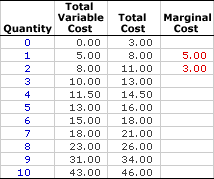Now, the marginal benefit curve has another name known as demand. Hence, the opportunity cost is factored in as a cost here. However, the first slice of pizza only cost you 30 rupees, but then the second slice of pizza is a higher cost, including the opportunity cost for making this. So, the marginal cost curve’s another name is supply.

### Marginal cost example

Let’s produce one thousand Radios. Now the production cost of one thousand radios is two lakh rupees. Next, when we produce one thousand one radios, the production cost will increase by two lakh one 50 rupees. Now the 150 rupees is the marginal cost. Moreover, it may also the aggregate of variable cost or prime cost plus variable overheads.

Radios, 1000 production cost = 2, 00,000 rupees.

Next, 1001 production cost = 2, 00,150 rupees.

Now, marginal cost = 150 rupees.

#### What is the marginal cost and example?

With the increase in one unit of output, the total cost increases, and the total increased unit is the marginal cost. However, marginal cost is a change in total cost by producing an additional unit of output. Suppose we produce five units which cost 150 rupees. Now when we produce six units, the cost will be 160 rupees. So the marginal cost will be 10 rupees.

#### What is the marginal cost of capital?

The marginal cost of capital means the cost of raising an additional rupee. That means we already have a balance that has share capitals, preference share, ventures and so on. If we need to increase the shares, then increasing the share is the marginal cost of capital.

#### What is a marginal benefit example?

For example, a person is willing to pay 20 rupees for a biscuit, so the marginal benefit of consuming the biscuit is 20 rupees.

#### What happens when marginal cost increases?

Moreover, the average cost goes up when marginal cost increases.

#### How is the total cost calculated?

So, TC (total cost) = TFC (total fixed cost) + TVC (total variable cost) is the formula for calculating the total cost.

How do I calculate marginal products?

(Q^n – Q^n-1) / (L^n – L^n-1) is the formula for calculating the marginal products.

#### Is WACC a marginal cost?

Yes, WACC is a marginal cost.